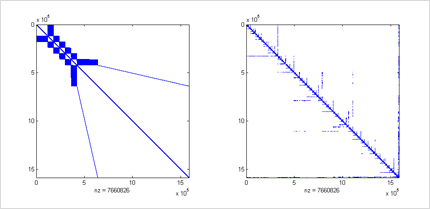19Dec/11Off

## Reordering to increase parallelism

by Kyle

Solving a dense triangular matrix (such as those produced by an LU factorization) is a serial process – each row must be solved before moving on to the next row. However, when solving a sparse triangular matrix, it’s possible to group these operations into levels where an entire level of unknowns can be solved in parallel before moving on to the next level. In the worst case, there are n levels and the entire process must be serialized. In the best case, there is one level and the entire process can be parallelized (this would only happen in a diagonal matrix).

As expected, one might seek to the decrease the number of levels in their sparse matrix in an effort to increase parallelism and (potentially) decrease the time it takes to solve their sparse triangular matrix. In many cases this can be achieved by reordering the sparse matrix. A matrix reordering simply involves swapping a number of rows and/or columns to alter the structure of the matrix while preserving the values. In many cases, reordering is performed to increase accuracy or reduce the fill-in of direct solve methods. However, in the case of CULA Sparse, we reorder the matrix to increase parallelism. In the sparse linear algebra domain, there exist a number of different reordering schemes (such as minimum degree, approximate minimum degree) but they are beyond the scope of this blog post.This image illustrates how matrix reordering is applied to a sparse matrix. In this case, a large circuit simulation problem (AMD/G3_circuit) is reordered using the symmetric minimum degree reordering method.

One of the options for CULA Spare’s ILU0 preconditioner is reordering. This will (potentially) reduce the levels in the lower and upper triangular factors produced by the factorization in an effort to increase the parallelism. Since applying the ILU0 preconditioner through two triangular solves is typically a massive bottleneck, any speed-up here will directly decrease the total time needed to converge on a solution.

When applying matrix reordering to a real world matrix such as the circuit simulation problem introduced above, we can decrease the number of levels from 2594 to 15. This decreases the time to solve the triangular matrixes from 24.2 ms to 8.5 ms - a 2.8x speedup! When using the reordered ILU0 preconditioner with the conjugate gradient solver, we see the total time per iteration drop to 53.4 ms to 22.1 ms – 2.4x speedup!

In conclusion, CULA Sparse’s ILU0 reordering option (more info) can be used to drastically reduce the time it takes to apply the triangular factors produced by the LU factorization. However, one must also consider that the reordering step has a steep calculation overhead. Additionally, since the structure of the matrix is changing, some of the conjugate-based methods will now take a different number of iterations to converge on a solution.